Editor Ratings:
User Ratings:
[Total: 0 Average: 0]

Here, are 5 free Financial Calculator apps for Android. Android has its own calculator which allows you to perform basic mathematical calculations on your Android phone. If you want to do some financial calculations in one go, then it becomes harder to perform calculation with basic calculator installed on your Android phone.

Google Play Store has come up with multiple Financial Calculator apps to perform financial calculations in one go. We will discuss some of these best free financial calculator apps.

## Financial Calculators: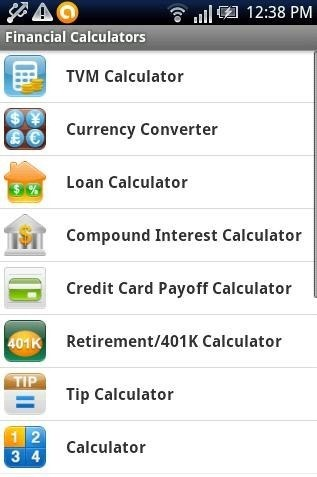Financial Calculators is a free Android app which provides an advanced calculator with the functionality to calculate all the finance related calculations. Financial calculator includes loan calculator, compound interest calculator, credit card payoff calculator, retirement calculator, tip calculator, normal/regular calculator, APR calculator, return on investment calculator, auto loan calculator, and more. The app is full fledge calculator app to calculate almost all types of financial calculations on Android. Financial Calculator makes it easy for you to make your calculation fast by just entering the values. You just have to launch the app, select the calculator, and you are ready to perform financial calculations on your Android phone. The calculator app also provides you the option to hide unwanted calculator from the long list of calculators in the app.

## Financial Calculator: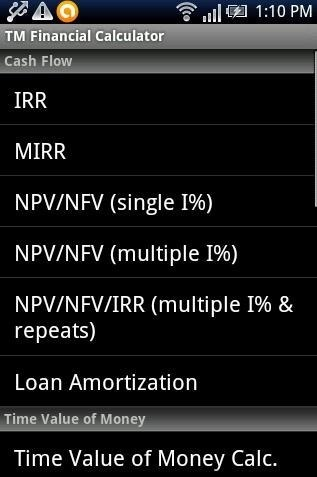Financial Calculator is a free Android app to perform financial calculations on Android. The calculators includes IRR calculator, MIRR calculator, NVP calculator, time value of money calculator, PV/FV simple interest calculator, PV of growing perpetuity, PV/FV of a growing annuity, effective rate converter, gamma & beta function, depreciation schedule calculator, tip calculator, bond calculator, and more. Financial Calculator app is a simple app that allows you to perform almost all the financial calculation with ease. It becomes faster to make financial calculation containing mathematical formulas and tricks. Normal/regular calculator of Android is unable to perform all these calculation which is directly related to your financial transaction at your home, in your office, or in your business.

## Smart Financial Calculator: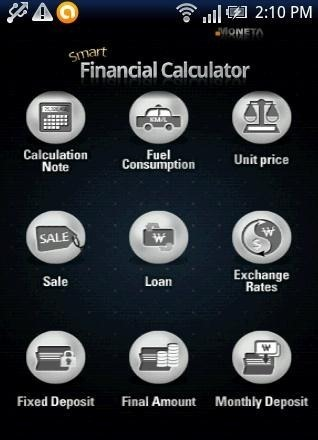Smart Financial Calculator is a free Android app to make financial calculations on Android. Smart Financial Calculator is a bit different financial calculator app for Android which includes different calculators as compared to 1st and 2nd calculator apps discussed above. The app allows you to prepare calculation note, fuel consumption calculator, unit price calculator, sale calculator, loan calculator, exchange rate calculator, and more. The app even has a smart feature to shake and delete the values entered or calculations done on Android. Just shake your Android phone and get ready for next calculation.

## Financial Calculator by Ulrich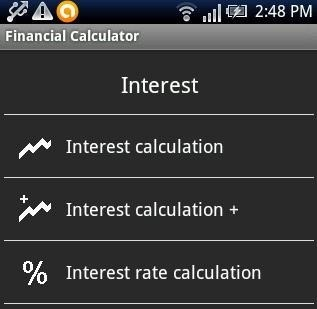Financial calculator by Ulrich is a free Android app to make basic financial calculations related to interest. The app totally deals with interest calculation. You can easily calculate any type of interest amount and interest rate on your Android phone. The calculator app also includes compound interest calculator and savings calculator. The app interface is really simple to understand and use. The calculation made by the app are very fast. You can save withholding tax and inflation rate to let the app make financial calculations accordingly. The good part of this financial calculator app is that the free version of the app is ads free in Google Play Store. While searching Financial calculator, you will find many calculators with the same name (financial calculator). Click the above link or check for financial calculator by Ulrich.

## Financial Calculators Lite: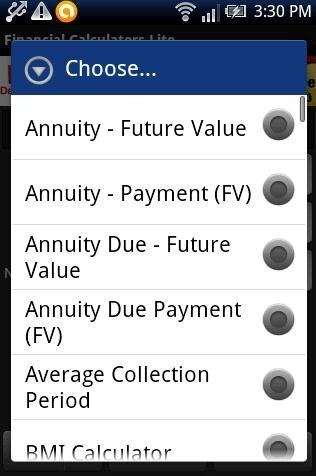Financial Calculators Lite is a free Android app which provides the bundle of calculators on Android. The app includes over 70+ financial calculators to calculate financial transactions on Android. This Android calculator app makes your work easy by proving the selection to the long list of calculators on your Android. Below are few which we thought were useful out of the long list of calculators provided by this Financial Calculators Lite app.

BMI Calculator calculator, Discount Calculator calculator, Loan – Payment calculator, Net Asset Value calculator, Price to Book Value calculator, Quick Ratio calculator, Rate Of Inflation calculator, Retention Ratio calculator, Return on Investment (ROI) calculator, Sales Tax Calculator calculator, Tip Calculator, Earnings Per Share calculator, Currency Converter, Compound Interest calculator, Capital Gains Yield calculator.

You can check calculator apps for Android reviewed by us.

If you find any other good financial calculator for Android, then do let us know in comments.

 Editor Ratings: User Ratings:[Total: 0 Average: 0] Works With: Android Free/Paid: Free Tags: android